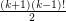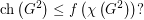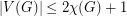# chromatic number

## Cycles in Graphs of Large Chromatic Number ★★

Author(s): Brewster; McGuinness; Moore; Noel

Conjecture   If, thencontains at leastcycles of length.

Keywords: chromatic number; cycles

## Erdős–Faber–Lovász conjecture ★★★

Author(s): Erdos; Faber; Lovasz

Conjecture   Ifis a simple graph which is the union ofpairwise edge-disjoint complete graphs, each of which hasvertices, then the chromatic number ofis.

Keywords: chromatic number

## Choosability of Graph Powers ★★

Author(s): Noel

Question  (Noel, 2013)   Does there exist a functionsuch that for every graph,## Ohba's Conjecture ★★

Author(s): Ohba

Conjecture   If, then.

## Bounding the chromatic number of triangle-free graphs with fixed maximum degree ★★

Author(s): Kostochka; Reed

Conjecture   A triangle-free graph with maximum degreehas chromatic number at most.

Keywords: chromatic number; girth; maximum degree; triangle free